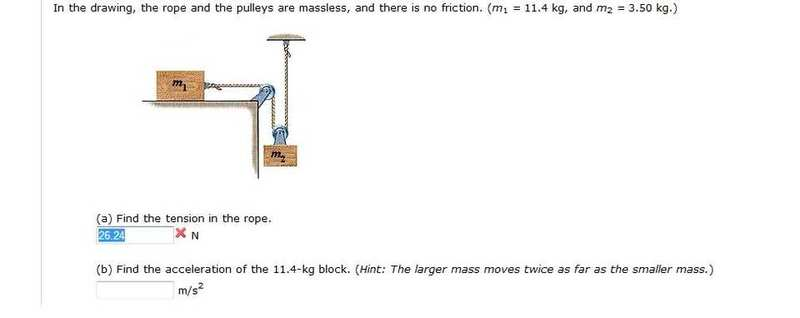# Help! Tension and Acceleration of 2 masses with a pulley!

drastice
Here is the## The Attempt at a Solution

Please teach me how to solve this
thanks!

drastice
Show your work and you'll get plenty of help.

You might want to read this: http://hyperphysics.phy-astr.gsu.edu/hbase/N2st.html#c1"

Well i don't have a lot of work to show.. I know
F=ma
The bottom one i believe would be *gravity (9.*8)
i'm just really lost on what equation i need to be using to get the tension in this setup

Last edited by a moderator:
Mentor
Start by looking over some of the problems in that link I gave you.

You'll need to draw free body diagrams for each mass, then apply Newton's 2nd law to each.

drastice
m2g-t=m2a
t=m1a

??
but i am missing acceleration and tension so how do i solve when i have 2 unknowns?

cupid.callin
you sure tension on m2 till be just T ?

and use the hint of question to find the acceleration of the blocks

Mentor
m2g-t=m2a
t=m1a
Almost. Fix your first equation using cupid.callin's hint.

but i am missing acceleration and tension so how do i solve when i have 2 unknowns?
Luckily you have two equations.

drastice
you sure tension on m2 till be just T ?

and use the hint of question to find the acceleration of the blocks

i'm not sure what you mean by that.. I'm looking at the link he gave me using the horrizontal pulley... Is this not the right equation? i don't see why you guys can't help me with the right equation

and for acceleration m2*gravity/m1+m2 = acceleration right??

Mentor
i'm not sure what you mean by that.. I'm looking at the link he gave me using the horrizontal pulley... Is this not the right equation?
That's a different problem. In your problem there's a pulley attached to the falling mass. Get the idea, don't just copy the equation.
i don't see why you guys can't help me with the right equation
We are helping. (But we're not going to do it for you.)
and for acceleration m2*gravity/m1+m2 = acceleration right??
No.

Don't skip steps. Fix your equation for the falling mass. Then you can solve for the acceleration and tension.

drastice
That's a different problem. In your problem there's a pulley attached to the falling mass. Get the idea, don't just copy the equation.

We are helping. (But we're not going to do it for you.)

No.

Don't skip steps. Fix your equation for the falling mass. Then you can solve for the acceleration and tension.

I don't expect you to do it for me... All i am asking is for the right equation.. I understand how to get acceleration i'm having trouble with the Tension..
i don't understand when he says "you sure tension on m2 till be just T ?"

Mentor
How many rope segments pull on mass 2?

drastice
Theres one pulling from mass1 and one in hte opposite direction from the ceiling..
How do i add this to the equation?

Mentor
What are all the forces acting on m2? Each rope segment applies its own tension.

drastice
What are all the forces acting on m2? Each rope segment applies its own tension.

it has gravity and tension from both ropes..
I have no idea how to add this to the equation though.

drastice
this problem is due in 18 minutes...
i just don't understand that equation.. You're not doing the problem for me be helping me with an equation... ;[

Mentor
it has gravity and tension from both ropes..
I have no idea how to add this to the equation though.
You don't 'add it' to the equation, you use it to come up with a correct equation. The approach is always the same: ΣF = ma.

We've been focusing on the forces on m2 (the ΣF part of the equation), but you are making a second error. You have called the acceleration of each mass 'a', but their accelerations are not the same. (See the hint given in your diagram.)

Homework Helper
i just don't understand that equation.. You're not doing the problem for me be helping me with an equation... ;[

Doing the problem for you would be against the rules. If you don't understand the help that is given to you, then you must learn more so you can understand it.